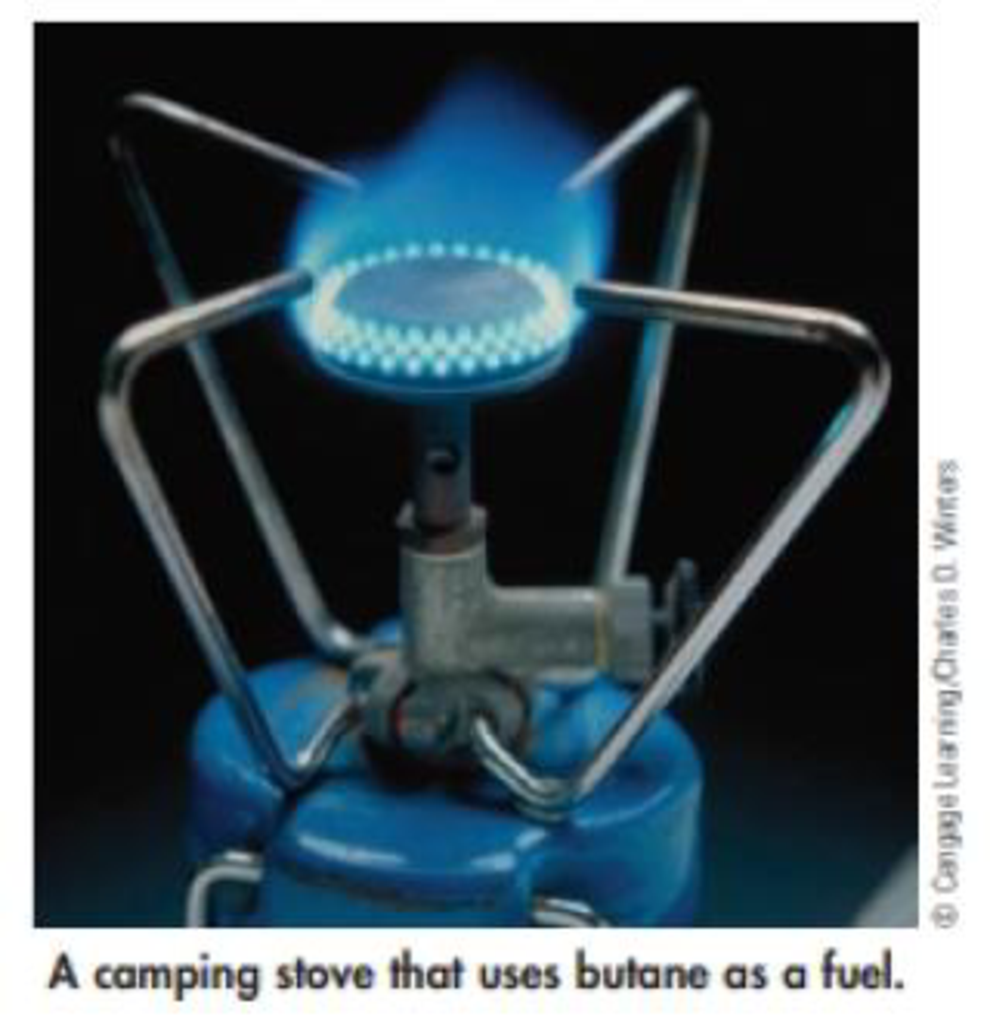Chapter 5, Problem 84GQ

Chapter
Section
Textbook Problem

Camping stoves are fueled by propane (C3H8), butane [C4H10(g), ΔfH° = –127.1 kJ/mol]. gasoline. or ethanol (C2H5OH). Calculate the enthalpy of combustion per gram of each of these fuels. [Assume that gasoline is represented by isooctane, C8H18(ℓ), with ΔfH° = –259.3 kJ/mol.) Do you notice any great differences among these fuels? How are these differences related to their composition?Interpretation Introduction

Interpretation:

The enthalpy of combustion per gram of propane and butane has to be calculated

Concept Introduction:

The standard enthalpy change of combustion of a compound is the enthalpy change which occurs when one gram of the compound is burned completely in oxygen under standard conditions, and with everything in its standard state

ΔrH0=ΣnΔfH0(products)-ΣnΔfH0(reactants)

The change in enthalpy, ΔH in kJ per mole of a given reactant for the reaction can be calculated as:

ΔrH=enthalpy changenumber of moles

ΔH=ΔrH×number of moles

Explanation

For propane the balanced equation is as follows:

C3H8+ 5 O23CO2+ 4 H2O

ΔrH0=[(3mol×-393.5kJ/mol)+(4mol×-285.83kJ/mol)]-[(1mol×-104.7 kJ/mol+0)]

ΔrH0=-2219.12kJ

The change in enthalpy, ΔH in kJ per mole of a given reactant for the reaction can be calculated as:

ΔrH=enthalpy changenumber of moles

ΔrH=-2219.12kJ/mol×1mol44.1g=-50.32J/g.K

So, the enthalpy of combustion is -50.32J/g

Still sussing out bartleby?

Check out a sample textbook solution.

See a sample solution

The Solution to Your Study Problems

Bartleby provides explanations to thousands of textbook problems written by our experts, many with advanced degrees!

Get Started